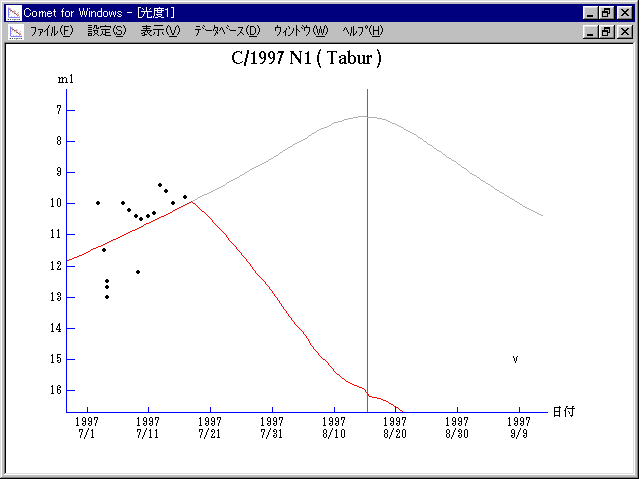# \$B%?%\$%P!

C/1997 N1 ( Tabur )###\$B%W%m%U%#!<%k(B

 \$BH/8+F|(B 1997\$BG/(B7\$B7n(B2\$BF|(B \$BH/8+8wEY(B 10\$BEy(B \$BH/8+ Vello Tabur (Wanniassa, Australian Capital Territory)

###\$B50F;MWAG(B

```   The following improved orbital elements by Kenji Muraoka,
are from 68 observations 1997 July 3 to 30, perturbations
by 9 Planets, Moon and 5 minor planets were taken into account.
The mean residual is +/- 0.82 arc seconds.

Epoch  =  1997 Aug. 20.0  TT       JDT = 2450680.5
T  =  1997 Aug. 15.47943       +/- 0.00058 (m.e.) TT
Peri. =  344.19149                +/- 0.00402
Node  =  147.61014                +/- 0.00275   (2000.0)
Incl. =   85.96427                +/- 0.00313
q  =    0.3956521              +/- 0.0000400 AU
e  =    1.0000476              +/- 0.0000786
1/a  =   -0.0001202              +/- 0.0001987 1/AU
```

###\$B@1?^(B1997\$BG/(B 4\$B7n(B12\$BF|!A(B1997\$BG/(B 8\$B7n(B10\$BF|(B1997\$BG/(B 8\$B7n(B10\$BF|!A(B1998\$BG/(B10\$B7n(B14\$BF|(B

###\$B8wEYJQ2=(B

```        m1 = 10.5 + 5 log\$B&\$(B + 10.0 log r  [   ,-28]  (              \$B!A(B1997\$BG/(B 7\$B7n(B18\$BF|(B)
m1 =  8.0 + 5 log\$B&\$(B - 18.0 log r  [-28,  0]  (1997\$BG/(B 7\$B7n(B18\$BF|!A(B1997\$BG/(B 8\$B7n(B15\$BF|(B)
m1 = 21.5 + 5 log\$B&\$(B + 15.0 log r  [  0,   ]  (1997\$BG/(B 8\$B7n(B15\$BF|!A(B              )

\$B"((B \$B3%?'\$N6J@~\$O!"(Bm1 = 10.5 + 5 log\$B&\$(B + 10.0 log r \$B\$N8wEY6J@~\$G\$9!#(B
```##### \$B50F;MWAG\$OB<2,7r<#;a\$N7W;;\$K\$h\$k\$b\$N\$G\$9!#(B \$B@1?^\$O(B StellaNavigator Ver.2.0 for Windows (\$B%"%9%H%m%"!<%D(B \$BJTCx(B / \$B%"%9%-!<=PHG6I4)(B) \$B\$G:n@.\$7\$?\$b\$N\$G\$9!#(B \$B8wEY%0%i%U\$O(BComet for Windows\$B\$G:n@.\$7\$?\$b\$N\$G\$9!#(B